# KSEEB Solutions for Class 6 Maths Chapter 12 Ratio and Proportion Ex 12.3

Students can Download Chapter 12 Ratio and Proportion Ex 12.3 Questions and Answers, Notes Pdf, KSEEB Solutions for Class 6 Maths helps you to revise the complete Karnataka State Board Syllabus and score more marks in your examinations.

## Karnataka State Syllabus Class 6 Maths Chapter 12 Ratio and Proportion Ex 12.3

Question 1.
If the cost of 7 m of cloth is Rs 294, Find the cost of 5 m of cloth.
Solution:
Cost of 7m cloth = Rs 294
Cost of 1 m cloth = $$\frac{294}{7}$$ = Rs 42
Therefore, Cost of 5m cloth = 42 × 5 = Rs 210

Question 2.
EKta earns Rs 1500 in 10 days. How much will she earn in 30 days?
Solution:
EKta earns money in 10 days = Rs 1500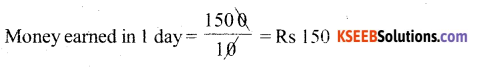Therefore, Money earned in 30 days = 150 × 30 = Rs 4500.

Question 3.
If it has rained 276 mm in the last 3 days. How many cm of rain will fall in one full week (7 days)? Assume that the rain continues to fall at the same rate.
Solution:
Measure of rain in 3 days = 276 mm
Measure of rain in 1 day = $$\frac{276}{3}$$ = 92 mm
Therefore, measure of rain in 7 days = 92 × 7 = 644 mmQuestion 4.
cost of 5 kg of Wheat is Rs 30.50,
a) What will be the cost of 8 kg of wheat?
b) What quantity of wheat can be purchased in Rs 61?
Solution:
a) Cost of 5 kg Wheat = Rs 30.50
Cost of 1kg Wheat = $$\frac{30.50}{5}$$ = Rs 6.10
Therefore, Cost of 8 kg Wheat = 6.10 × 8 = Rs. 48.80

b) Wheat purchase in Rs 30.50 = 5 kg
Wheat purchase in Rs 1 = $$\frac{5}{30.50}$$ kg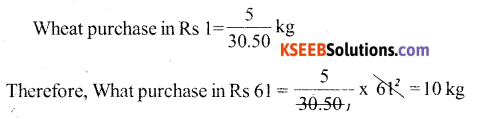Question 5.
The temperature dropped 15 degree Celsius in the last 30 days. If the rate of temperature drop remains the same, how degree will the temperature drop in the next ten days?
Solution:
Temperature drop in 30 days = 15°c
Temperature drop in 1 days = $$\frac{15}{30}=\left(\frac{1}{2}\right)^{\circ} \mathrm{C}$$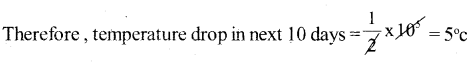Thus, these will be a temperature drop of 5°c on the next ten days.Question 6.
Shaina pays Rs 7500 as rent for 3 months. How much does she has to pay for a Whole year, if the rent per month remains same?
Solution:
Rent for 3 months = Rs 7500
Rent for 1 months = $$\frac{7500}{3}$$ = Rs 2500
Therefore, rent for 12 months = 2500 × 12 = 30.000
Thus, she has to pay Rs 30.000 for a whole year.

Question 7.
Cost of 4 dozens bananas is Rs 60. How many bananas can be purchase for Rs 12.50?
Solution:
Numbers of bananas bought in Rs 60 = 4 × 12 = 48
Numbers of bananas bought in Rs 1 = $$\frac{48}{60}$$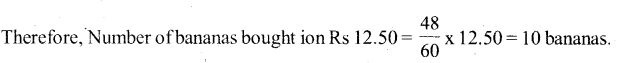Thus, 10 bananas can be purchase for Rs. 12.50Question 8.
The weight of 72 books is 9 kg. What is the weight of 40 such books?
Solution:
Weight of 72 books = 9 kg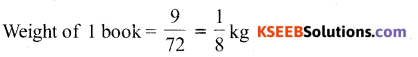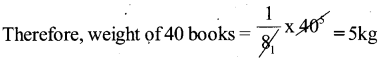Thus, the weight of 40, Such books is 5 kg

Question 9.
A truck requires 108 litres of diesel for covering a distance of 594 km. How much diesel will be required by the truck to cover a distance of 1650 km?
Solution:
Diesel required for 594 km = 108 litres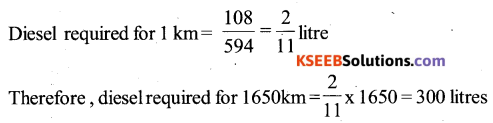Thus, 300 litres diesel will be required by the truck to cover a distance of 1650 kmQuestion 10.
Raju purchases 10 pens for Rs 150 and manish buys 7 pens for Rs 84. Can you say Who got the pens cheaper?
Solution:
Raju purchase 10 pens for Rs 150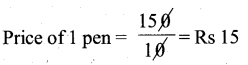Manish purchase 7 pens for Rs 84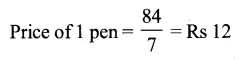Therefore, manish got the pens cheaperQuestion 11.
Anish made 42 runs in 6 overs and an up made 63 runs in 7 overs. Who made more runs per over?
Solution:
Runs made by Anish in 6 overs = 42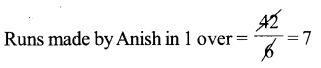Runs made by Anup in 7 overs = 63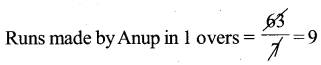Clearly Anup made more runs per over.